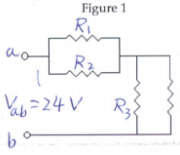# Problem: The resistors in the circuit shown in Figure 1 each have a resistance of 700 Ω.Find (a) the equivalent resistance of the circuit and (b) the current flowing through each resistor R if the input voltage is 24 volts.

🤓 Based on our data, we think this question is relevant for Professor Li's class at FIU.

###### Problem Details

The resistors in the circuit shown in Figure 1 each have a resistance of 700 Ω.Find (a) the equivalent resistance of the circuit and (b) the current flowing through each resistor R if the input voltage is 24 volts.